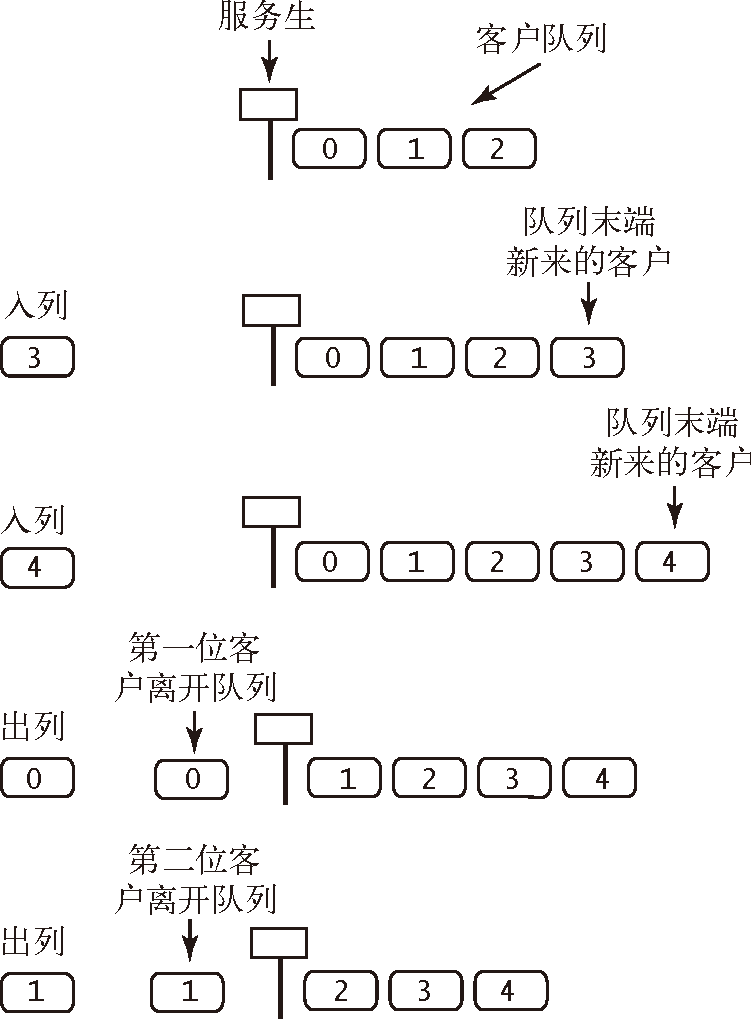# 算法（4th ed）(120)：基础——背包、队列和栈 5.1.5(API：先进先出队列）public static int[] readInts(Stringname){   In in = new In(name);   Queue<Integer> q = newQueue<Integer>();   while (!in.isEmpty())       q.enqueue(in.readInt());    int N = q.size();   int[] a = new int[N];   for (int i = 0; i < N; i++)      a[i] = q.dequeue();   return a;}
Queue 的用例